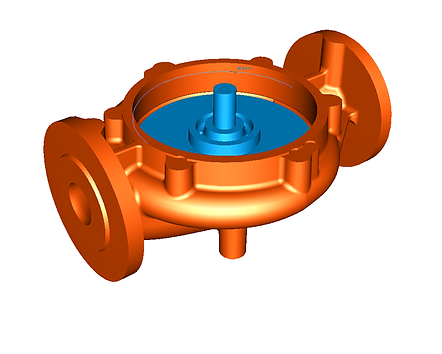## TURBOMACHINES

Projects/TurbomachinesSubmersible Pump

In the project, a submersible pump was examined with CFD analysis, and pump curves (Head-Flow, Power-Flow, Efficiency-Flow) curves were obtained.# .

Fire Pump

In the project, a fire pump with a suction diameter of 250 mm and an outlet diameter of 80 mm was examined with CFD analysis, pump curves (Height-Flow, Power-Flow, Efficiency-Flow) curves were obtained and NPSH calculations were made.# .

Fire Pump

In the project, a fire pump with a suction diameter of 315 mm and an outlet diameter of 80 mm was examined with CFD analysis, the curves of the pump (Head-Flow, Power-Flow, Efficiency-Flow) curves were obtained and NPSH calculations were made.# .

Fire Pump

In the project, a fire pump with a suction diameter of 250 mm and an outlet diameter of 100 mm was examined with CFD analysis, and the curves of the pump (head-flow, power-flow, efficiency-flow) were obtained.  NPSH was calculated.# .

Turbo Hydraulic Coupling

In the project, a turbo-hydraulic coupling was examined with CFD analysis, and the drive shaft (pump) and output shaft (turbine)  depending on the rotation speed,  torque and power calculations  calculated.# .

"In-Line" Centrifugal Pump

In the project,  By examining an "in-line" centrifugal pump with CFD analysis, pump curves (Head-Flow, Power-Flow, Efficiency-Flow) curves are obtained.  NPSH was calculated.# .

Submersible Pump

In the project, a submersible pump was examined with CFD analysis, and pump curves (Head-Flow, Power-Flow, Efficiency-Flow) curves were obtained, NPSH was calculated.# .

Mixed Flow Pump

In the project, a mixed-flow pump was examined with CFD analysis and pump curves (Head-Flow, Power-Flow, Efficiency-Flow) curves were obtained.  NPSH was calculated.# .

Submersible Pump

In the project, a stainless steel submersible pump was examined with CFD analysis and pump curves (Head-Flow, Power-Flow, Efficiency-Flow) curves were obtained. NPSH was calculated.# .

Centrifugal Pump

In the project, a centrifugal pump was examined with CFD analysis and pump curves (Head-Flow, Power-Flow, Efficiency-Flow) curves were obtained.  NPSH was calculated.# .

Centrifugal Pump

In the project, a centrifugal pump was examined with CFD analysis and pump curves (Head-Flow, Power-Flow, Efficiency-Flow) curves were obtained.  NPSH was calculated.# .

Watermeter

In the project, a water meter was examined with CFD analysis and the water flow rate was determined.  The watermeter rotational speeds were calculated.# .

Industrial Centrifugal Fan

In the project, a double suction industrial centrifugal fan with a diameter of 2400 mm  By examining with CFD analysis, fan curves (Discharge-Flow, Power-Flow, Efficiency-Flow) curves were obtained.  has been done.# .

Industrial Centrifugal Fan

In the project, 1200 mm diameter  an industrial centrifugal fan  By examining with CFD analysis, fan curves (Discharge-Flow, Power-Flow, Efficiency-Flow) curves were obtained.  has been done.# .

"In-Line" Fan

In the project, an "in-line" fan was examined with CFD analysis, and curves of the fan curves (Discharge Head-Flow, Power-Flow, Efficiency-Flow) curves were obtained.  has been done.